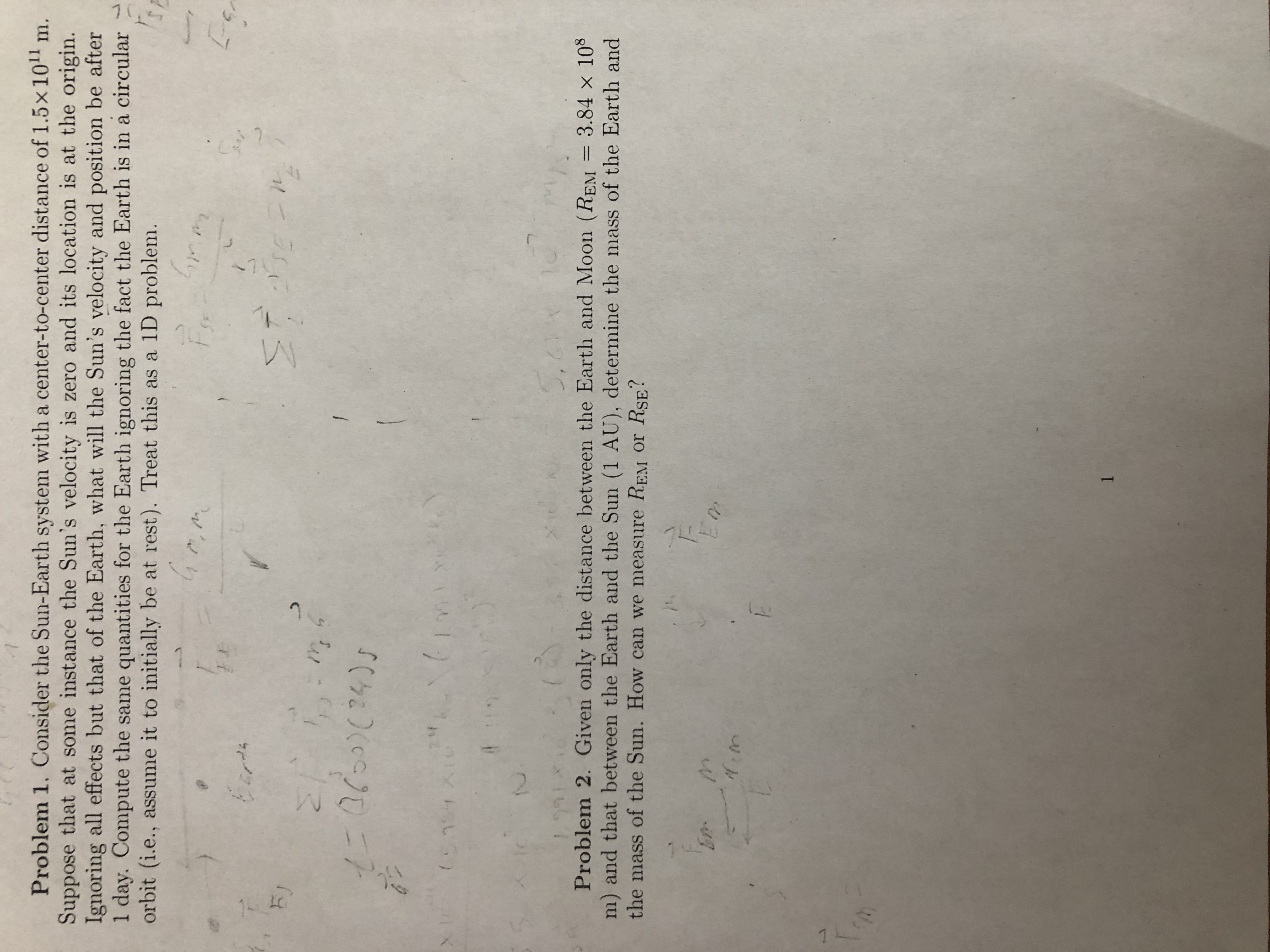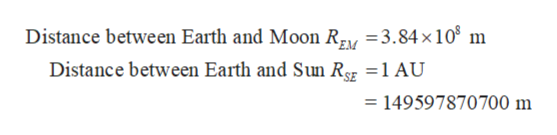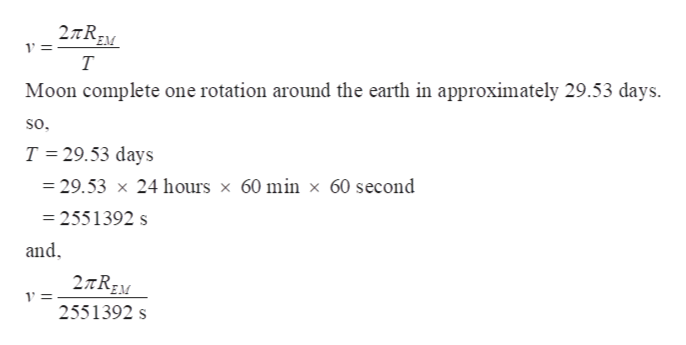# Problem 1. Consider the Sun-Earth system with a center-to-center distance of 1.5x 101 m.Suppose that at some instance the Sun's velocity is zero and its location is at the origin.Ignoring all effects but that of the Earth, what will the Sun's velocity and position be after1 day. Compute the same quantities for the Earth ignoring the fact the Earth is in a circularorbit (i.e., assume it to initially be at rest). Treat this as a 1D problem.GrEcrokFJ2/A2 57(5.994 Xt0495,21 10Problem 2. Given only the distance between the Earth and Moon (REM = 3.84 x 108m) and that between the Earth and the Sun (1 AU), determine the mass of the Earth andthe mass of the Sun. How can we measure REM or RSE?M11421 k(P

Question
36 views

I need help with problem 2 please.help_outlineImage TranscriptioncloseProblem 1. Consider the Sun-Earth system with a center-to-center distance of 1.5x 101 m. Suppose that at some instance the Sun's velocity is zero and its location is at the origin. Ignoring all effects but that of the Earth, what will the Sun's velocity and position be after 1 day. Compute the same quantities for the Earth ignoring the fact the Earth is in a circular orbit (i.e., assume it to initially be at rest). Treat this as a 1D problem. Gr Ecrok FJ 2/A 2 57 (5.994 Xt0 49 5,21 10 Problem 2. Given only the distance between the Earth and Moon (REM = 3.84 x 108 m) and that between the Earth and the Sun (1 AU), determine the mass of the Earth and the mass of the Sun. How can we measure REM or RSE? M 1 142 1 k (P fullscreen
check_circle

Step 1

Given,help_outlineImage TranscriptioncloseDistance between Earth and Moon Rzy 3.84x108 m Distance between Earth and Sun Rg =1 AU = 149597870700 m fullscreen
Step 2

Let the mass of moon and earth is mM and mE respectively. The moon is resolving around the earth so by the Newton’s law of gravitation:

Step 3

Now, Moon’s velocity is tota...help_outlineImage Transcriptionclose2TREN 1= T Moon complete one rotation around the earth in approximately 29.53 days. so. T 29.53 days 29.53 x 24 hours x 60 min x 60 second 2551392 s and 1' = 2551392 s fullscreen

### Want to see the full answer?

See Solution

#### Want to see this answer and more?

Solutions are written by subject experts who are available 24/7. Questions are typically answered within 1 hour.*

See Solution
*Response times may vary by subject and question.
Tagged in

### Gravitation